Electric Potential Energy Electric Potential DifferenceVoltage Physics Mr

• Slides: 18-Electric Potential Energy -Electric Potential Difference(Voltage) Physics Mr. Berman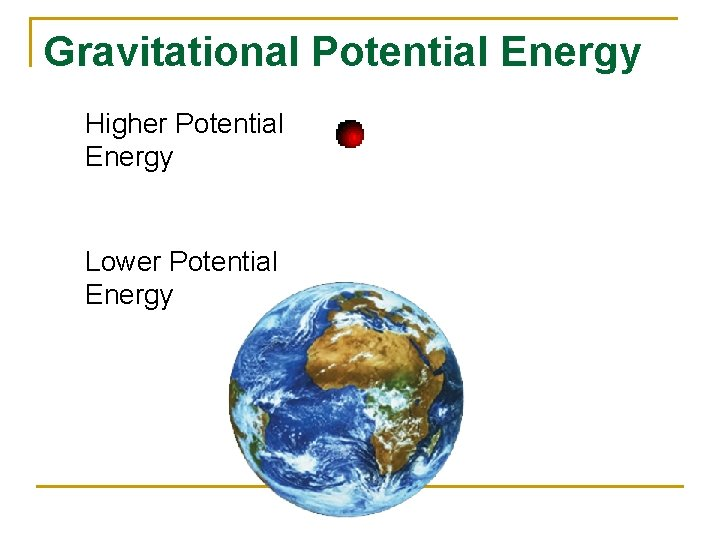Gravitational Potential Energy Higher Potential Energy Lower Potential Energy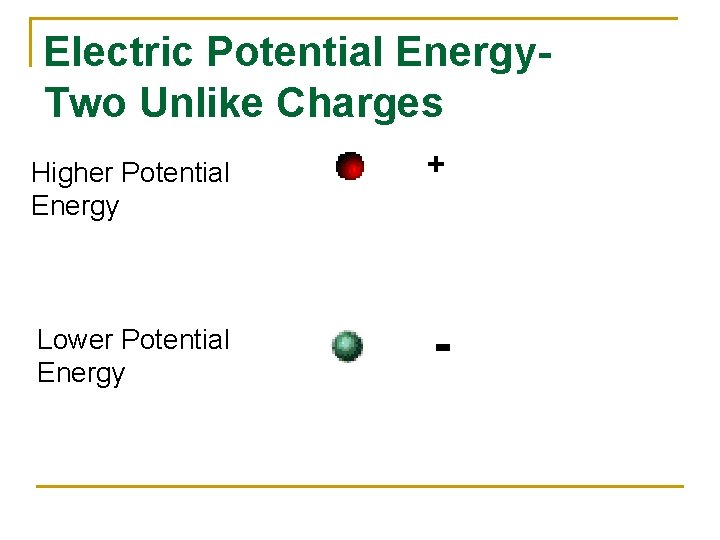Electric Potential Energy. Two Unlike Charges Higher Potential Energy + Lower Potential Energy -Work done by an Electric Force For a point charge, qo , in field, E: n Force from the field: F=qo. E n The work done by the force to move the charge from point A to B (in the direction of the field) is: W=Fd n The object loses potential energy.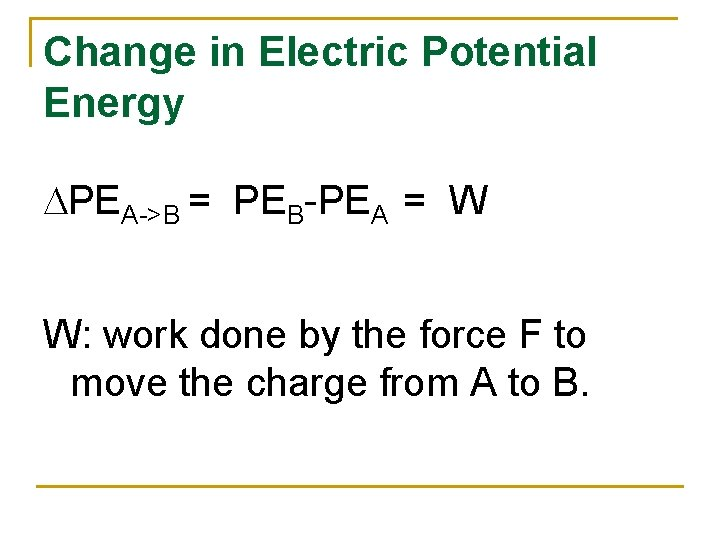Change in Electric Potential Energy DPEA->B = PEB-PEA = W W: work done by the force F to move the charge from A to B.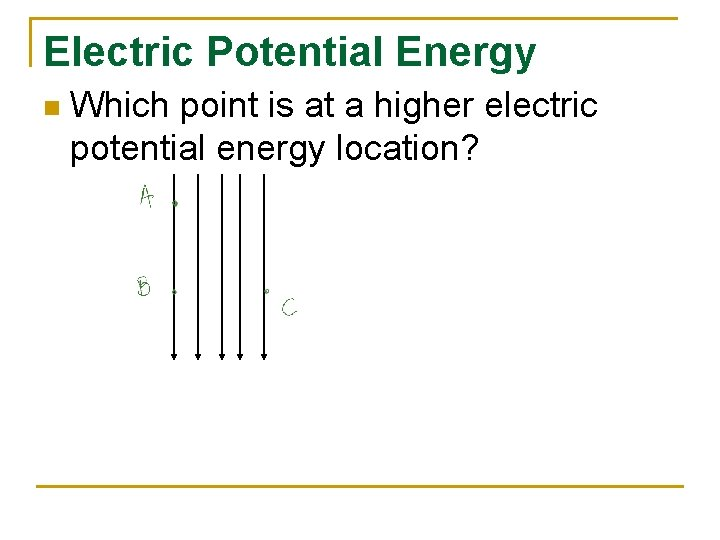Electric Potential Energy n Which point is at a higher electric potential energy location?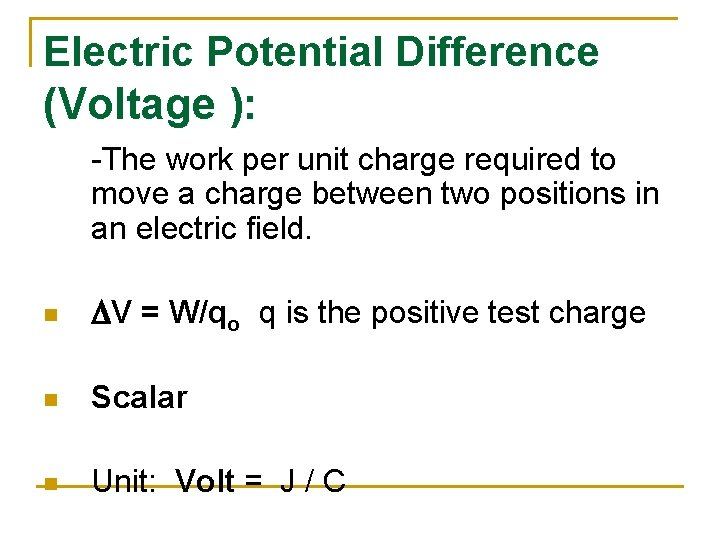Electric Potential Difference (Voltage ): -The work per unit charge required to move a charge between two positions in an electric field. n DV = W/qo q is the positive test charge n Scalar n Unit: Volt = J / C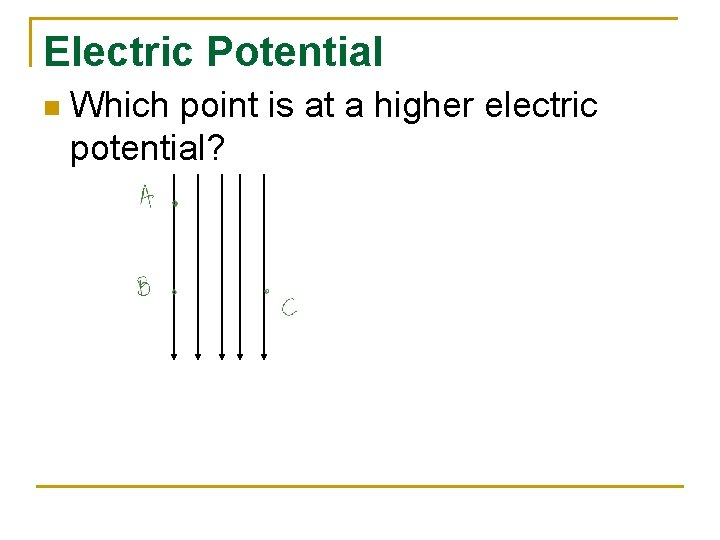Electric Potential n Which point is at a higher electric potential?Electric Potential n Electric Field lines point to the lower potential.Equipotential Lines Simulation of Field with Equipotential Lines http: //glencoe. mcgrawhill. com/sites/0078458137/student_view 0/chapter 21/elec tric_fields_applet. htmlElectric Field Strength (Vector) in Terms of Voltage n E= V/d Unit : V / m N/C Note: -Electric Field is a vector. -Voltage is a scalar. n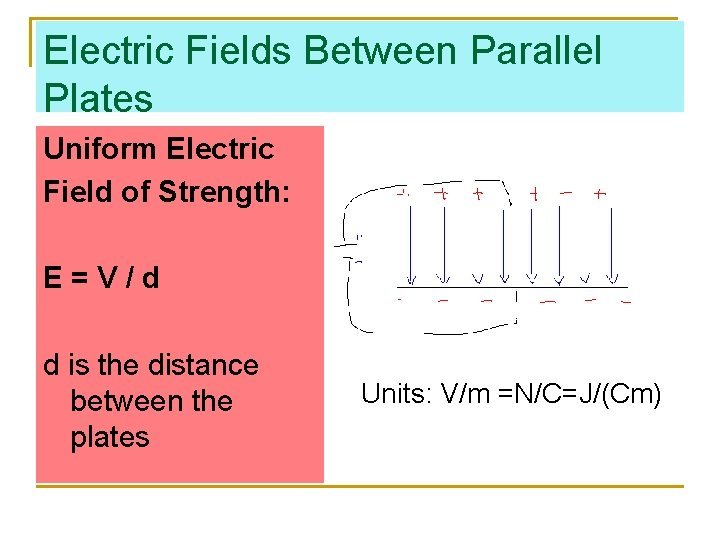Electric Fields Between Parallel Plates Uniform Electric Field of Strength: E=V/d d is the distance between the plates Units: V/m =N/C=J/(Cm)Force Experienced by a Test Charge Inside the Parallel Plate Field The field is uniform so at any point in the field a test charge would feel a force F = q. E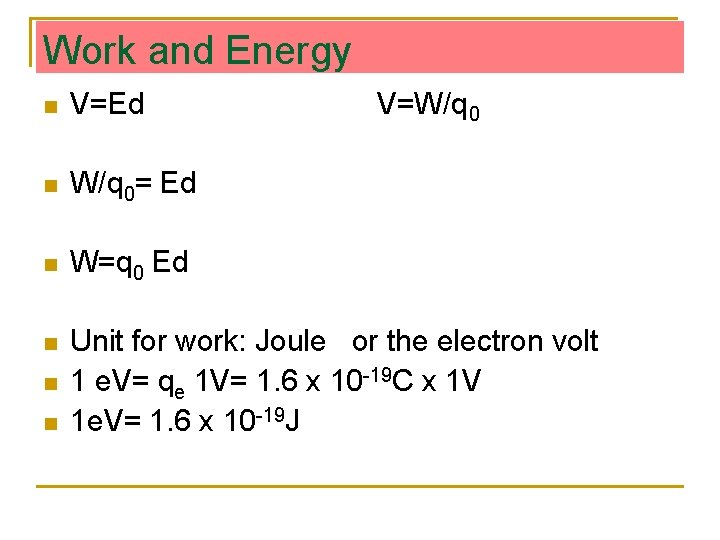Work and Energy n V=Ed n W/q 0= Ed n W=q 0 Ed n Unit for work: Joule or the electron volt 1 e. V= qe 1 V= 1. 6 x 10 -19 C x 1 V 1 e. V= 1. 6 x 10 -19 J n n V=W/q 0Find: a)The field inside the plates. b)The force felt by a 9 n. C charge that is situated in the field. Problem 1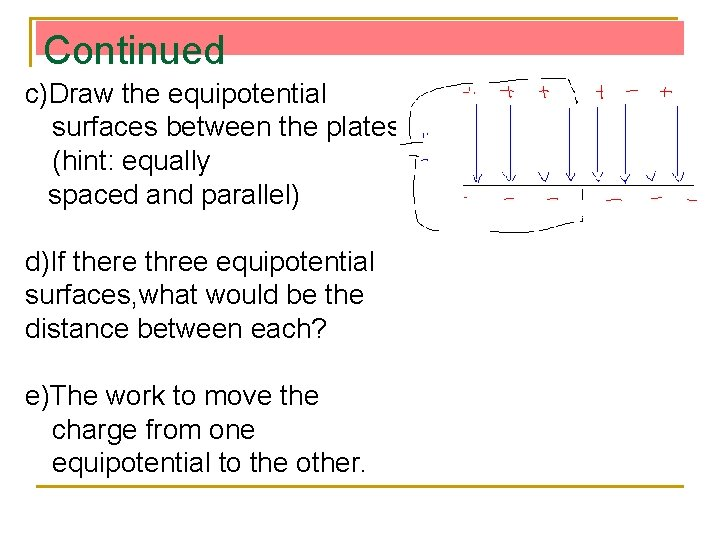Continued c)Draw the equipotential surfaces between the plates (hint: equally spaced and parallel) d)If there three equipotential surfaces, what would be the distance between each? e)The work to move the charge from one equipotential to the other.Continued f)The potential difference between each equipotential surface and the negative plate.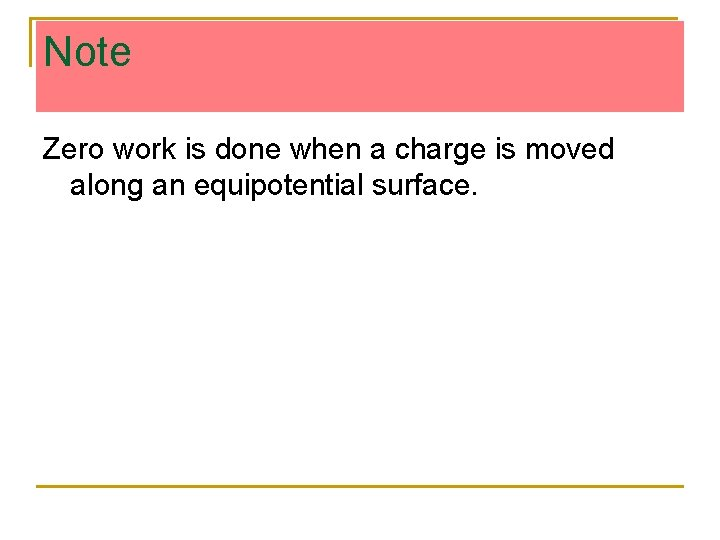Note Zero work is done when a charge is moved along an equipotential surface.